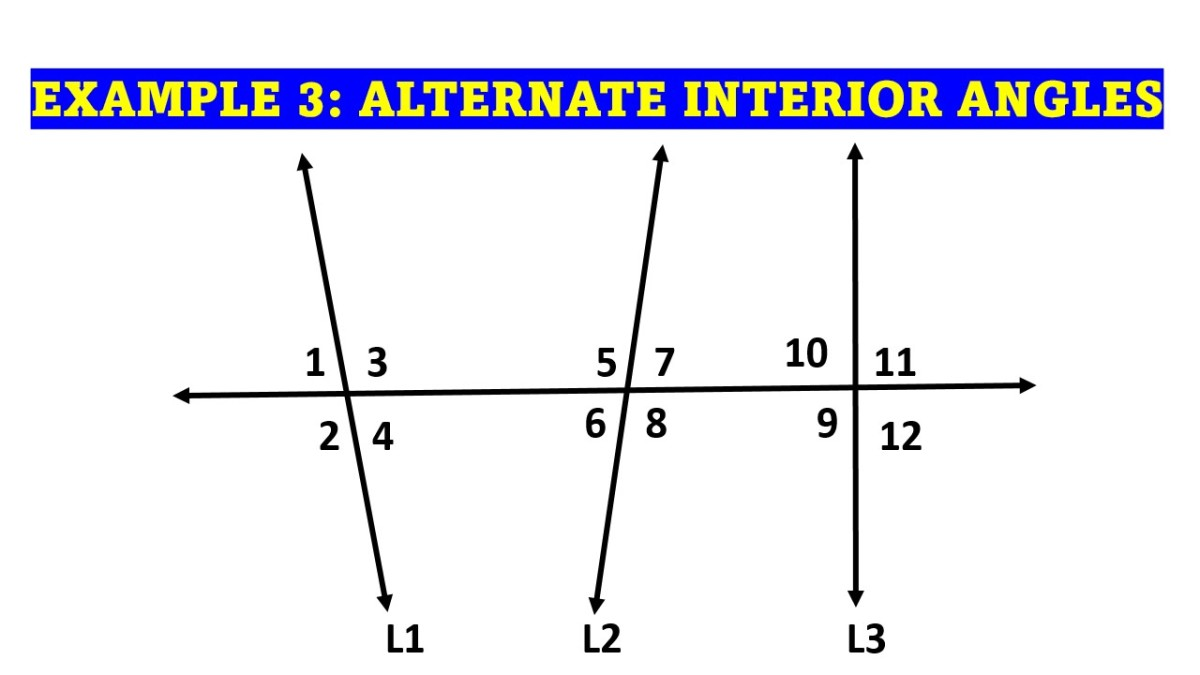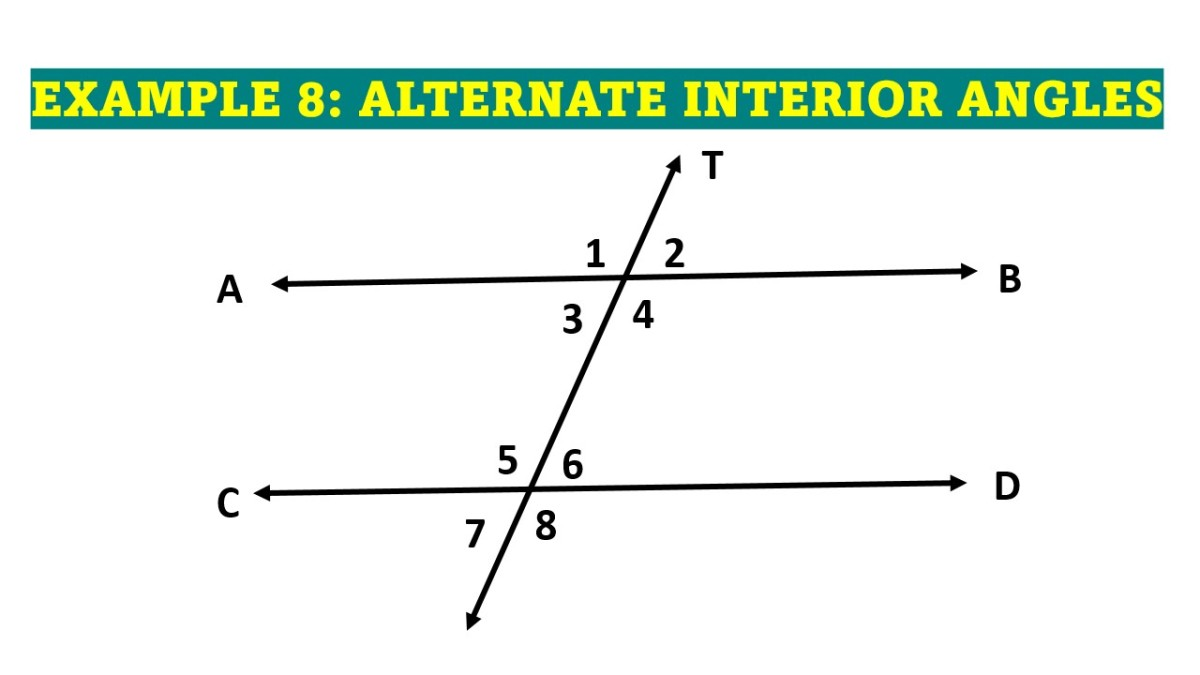# Alternate Interior Angles (Theorem and Examples)

• Author:
• Updated date:

Ray is a licensed engineer in the Philippines. He loves to write about mathematics and civil engineering.

## What Are Alternate Interior Angles?

Alternate interior angles are a pair of nonadjacent interior angles that are on opposite sides of the transversal. Given a transversal T of two coplanar lines, L1 and L2 must necessarily lie in the same plane that contains L1 and L2. It is because transversal T contains two distinct points on the plane. The figure below shows that line T is a transversal of lines L1 and L2. From the shown set of angles, angles 3, 4, 5, and 6 are called alternate interior angles.

## How Do You Spot Alternate Interior Angles?

In geometry, alternate interior angles are interior angles on opposite sides of the transversal. To spot alternate interior angles, look for a Z-shaped figure, as shown in the following figure. Alternate interior angles of two lines cut by a transversal are nonadjacent angles between the two lines and on opposite sides of the transversal.

## Alternate Interior Angles Theorem

The alternate interior angle theorem states that if two parallel lines are cut by a transversal, then alternate interior angles are congruent.

### Alternate Interior Angles Theorem Proof

Let L1 and L2 be parallel lines cut by a transversal T such that ∠1 and ∠3 are alternate interior angles. Let us prove that ∠1 ⩭ ∠3.

Alternate Interior Angles Theorem Proof

StatementsReasons

1. ∠1 ⩭ ∠2

1. PCA Postulate

2. ∠2 and ∠3 form vertical angles

2. Vertical Angles Definition

3. ∠2 ⩭ ∠3

3. Vertical Angle Theorem

4. ∠1 ⩭ ∠3

4. Transitive Property of Congruence

## The Converse of Alternate Interior Angles Theorem

The converse of the alternate interior angle theorem states that if two lines are cut by a transversal so that a pair of alternate interior angles are congruent, then the lines are parallel.

### The Converse of Alternate Interior Angles Theorem Proof

Let L1 and L2 be two lines cut by a transversal T such that ∠2 and ∠3 form congruent alternate interior angles as shown in the figure. Let us prove that L1 // L2.

The Converse of Alternate Interior Angles Theorem Proof

StatementsReasons

1. ∠3 and ∠1 are vertical angles, then ∠3 ⩭ ∠1.

1. Definition of Vertical Angles

2. Given ∠2 ⩭ ∠3, then ∠2 is also congruent to ∠1.

2. Transitive Property of Congruence

3. L1 // L2

3. Converse of PCA Postulate

Here are some alternate interior angles examples to practice on.

## Example 1: Identifying the Alternate Interior Angles

Name a pair of alternate interior angles in the picture below.

Scroll to Continue

By observing the given figure of lines cut by a transversal, then alternate interior angles are ∠4 and ∠6.

## Example 2: Naming Pairs of Alternate Interior Angles

In the figure below, line PQ is transversal to AB and CD. Name all pairs of alternate interior angles.

By observation, the alternate interior angles are ∠BPQ and ∠PQC, ∠PQD, and ∠APQ.

## Example 3: Example of Alternate Interior Angles With Three Lines Cut by a Transversal

In the figure below, name two pairs of alternate interior angles for lines

1. L1 and L3
2. L2 and L3Example of Alternate Interior Angles With Three Lines Cut by a Transversal

The given complex figure is quite tricky for starters, but if you will just stick to the alternate interior angles definition, then you can never go wrong. For lines L1 and L3, the alternate interior angles are angles 3, 9, and 4, 10. On the other hand, for L2 and L3, the alternate interior angles are angles 8, 10, and 7, 9.

## Example 4: Example of Alternate Interior Angles

Given L1 and L2 cut by a transversal L3, identify the value of x, ∠a, and ∠c shown.

### Solution

Given that L1 and L2 are parallel lines, we can conclude that the angles a and c are equivalent or equal. Thus, a = c.

a = c

5x - 18 = 3x + 20

5x - 3x = 20 + 18

2x = 38

x = 19

Substitute the x = 19 to the equations of a and c in terms of x.

∠a = 5x - 18

∠a = 5(19) - 18

∠a = 77

∠c = 3x + 20

∠c = 3(19) + 20

∠c = 77

Therefore, the value of x is 19, and ∠a and ∠c are both equal to 77.

## Example 5: Alternate Interior Angles Theorem Problem

In the figure below, L1 // L2 and L2 // L3. If ∠1 = x + 2y, ∠2 = 106, ∠3 = 3x - y, and ∠4 = 115, find the values of x and y.

### Solution

Since L1 // L2 and L2 // L3, then the measures of alternate interior angles are equal. In particular, we have the following solution.

∠1 = ∠2

x + 2y = 106

∠3 = ∠4

3x - y = 115

Rewrite the obtained equations and treat them as a system of linear equations. After obtaining the value of y, substitute it for any of the two equations.

-3(x + 2y = 106)-3

3x - y = 115

-3x -6y = -318

3x - y = 115

-7y = - 203

y = 29

x + 2y = 106

x + 2(29) = 106

x = 48

The values of x and y are 48 and 29, respectively.

## Example 6: Converse of Alternate Interior Angles Theorem Problem

Use the given information to determine which lines in the figure below are parallel.

1. ∠BDE ≅ ∠IHD
2. ∠IBD ≅ ∠BDE

1. Angles BDE and IHD are corresponding angles formed when lines L3 and L4 are cut by transversal L2. Since they are congruent, then L3 is parallel to L4.
2. Angles IBD and BDE are alternate interior angles formed when lines L1 and L2 are cut by the transversal L3. Since they are congruent, then L1 is parallel to L2.

## Example 7: Proving Conditions Using Alternate Interior Angle Theorem

Given that AB is parallel to DE, and ∠BAD is congruent to ∠ABD. Prove that ray DE bisects ∠BDC.

### Solution

To prove that the ray DE bisects ∠BDC, we need to show that ∠CDE ⩭ ∠EDB. Please see the following table of statements and reasons.

Proving Conditions Using Alternate Interior Angle Theorem

StatementsReasons

1. AB // DE

1. Given

2. PCA Postulate

3. ∠ABD ⩭ ∠EDB

3. Alternate Interior Angle Theorem

4. Given

5. ∠CDE ⩭ ∠ABD

5. Transitive Property of Congruence

6. Transitive Property of Congruence

7. DE bisects ∠BDC

7. Definition of Angle Bisector

Therefore, DE bisects ∠BDC.

## Example 8: Alternate Interior Angles in a Parallel Line Cut by a Transversal

Use the diagram shown below and the given information to solve the alternate interior angles example. Given that parallel lines AB and CD are cut by a transversal, find the following.

a. In which of the following angles is ∠3 congruent given that they are alternate interior angles?

b. Given m∠4 = 125, find the degree measure of ∠5.

c. Given m∠4 is represented by 5x - 80 and m∠5 is represented by 2x - 5, solve for x.

d. Given m∠3 is represented by ½ x + 25 and m∠6 is represented by 4x - 45, find the value of x.Alternate Interior Angles in a Parallel Line Cut by a Transversal

a. When parallel lines are cut by a transversal, alternate interior angles are formed. If you look for a z-shape, you can find the alternate interior angles at the two corners inside the z. Alternate interior angles, such as m∠3 and m∠6, are congruent.

b. Alternate interior angles are congruent, so m∠4 and m∠5 are congruent.

c. The angles 4 and 5 are alternate interior angles. Alternate interior angles are congruent, so set their measures equal to each other and solve for x.

5x - 80 = 2x - 5

3x - 80 = - 5

3x = 75

x = 25

d. Angles 3 and 6 are alternate interior angles. They are congruent, so set the measures equal to each other and solve for x.

½ x + 25 = 4x - 45

25 = 3.5x - 45

70 = 3.5x

x = 20

## Example 9: Congruent Alternate Interior Angles

Given the figure below, r // s and transversal l. Find the value of x and y.
m∠3 = 4x + y
m∠5 = x + 5y
m∠6 = 5x - 2y

### Solution

Analyzing the given figure, we can say that ∠3 and ∠6 are congruent alternate interior angles. Also, ∠3 and ∠5 are supplementary angles. These facts lead to the following system of equations.

∠3 = ∠6

4x + y = 5x - 2y

4x - 5x = -2y - y

-x = -3y

x - 3y = 0

∠3 + ∠5 = 180

(4x + y) + (6x + 5y) = 180

10x + 6y = 180

Divide each term of the second equation by two.

½ (10x + 6y) = (180) ½

5x + 3y = 90

Simplify the two equations using addition. Then, substitute 15 for x into the equation x - 3y = 0.

x - 3y = 0

5x + 3y = 90

6x = 90

x = 15

x - 3y = 0

15 - 3y = 0

-3y = -15

y = 5

The solution results in x = 15 and y = 5. Substitute these values to the equations given.

m∠3 = 4(15) + 5

m∠3 = 65°

m∠5 = 15 + 5(5)

m∠5 = 115°

m∠6 = 5(15) - 2(5)

m∠6 = 65°

Are alternate interior angles congruent?

Alternate interior angles are not always congruent. Congruent alternate interior angles are angles produced by a transversal intersecting two parallel lines. In which, in alternate interior angles, two lines cut by a transversal may not always be parallel. See the examples shown above.

Are alternate interior angles supplementary?

Alternate interior angles are not always supplementary. They can be supplementary if two parallel lines are cut by a transversal. But most of the time, they are supplementary.

What is the alternate interior angles definition?

Alternate interior angles are the angles formed when a transversal intersects two coplanar lines.

What are some examples of alternate interior angles in real life?

Some of the examples of alternate interior angles in real life are line rail transits intersections, window frames, roadway intersections, fire exit stairways, road traffic paints, etc.

This content is accurate and true to the best of the author’s knowledge and is not meant to substitute for formal and individualized advice from a qualified professional.